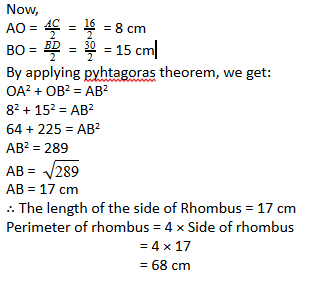# NCERT Solutions class 7 Maths Chapter-6 Exercise 6.5

NCERT Solutions Class-7 Maths chapter-6 Triangle and its properties Exercise-6.5 is prepared by academic team of pw all the questions of NCERT text book are solved step by step with proper and detail solutions explaining each and every questions . For More and additional questions of CBSE class 7 maths you can go to class 7 maths sections. NCERT class 7 Maths Solutions is the best way to enhanced your mathematics skill. And pw practice worksheet & question bank will help you a lot .

## NCERT Solutions class 7 Maths Chapter-6 Triangle and its properties

### Solutions of Chapter Triangle and its properties Exercise-6.5

Question 1:
PQR is a triangle, right-angled at P.  If PQ = 10 cm and PR = 24 cm, find QR.

It is given in the question that,
PQ = 10 cm
Also,
PR = 24 cm
We have to find QR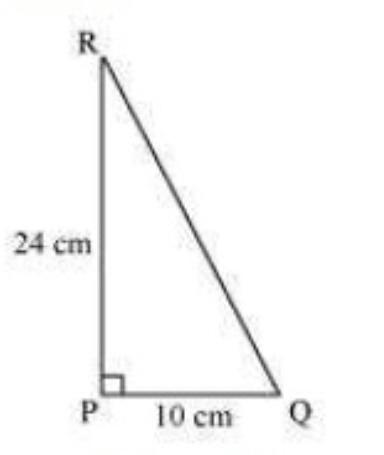We know that,
According to Pythagoras theorem,
In a right angled triangle:
(Hypotenuse)2 = (Perpendicular)2 + (Base)2
By applying Pythagoras theorem in the given question, we get:
(QR)2 = (PQ)2 + (PR)2
(QR)2 = (10)2 + (24)2
(QR)2 = 100 + 576
(QR)2 = 676
Hence,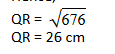Question 2:
ABC is a triangle, right-angled at C.  If AB = 25 cm and AC = 7 cm, find BC.

It is given in the question that,
AB = 25 cm
Also,
AC = 7 cm
We have to find BC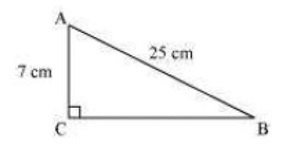We know that,
According to Pythagoras theorem,
In a right angled triangle:
(Hypotenuse)2 = (Perpendicular)2 + (Base)2
By applying Pythagoras theorem in ∆ABC, we get:
(AB)2 = (AC)2 + (BC)2
(BC)2 = (AB)2 - (AC)2
(BC)2 = (25)2 – (7)2
(BC)2 = 625 – 49
(BC)2 = 576
Hence,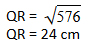Question 3:
A 15 m long ladder reached a window 12 m high form the ground on placing it against a wall
at a distance a.  Find the distance of the foot of the ladder from the wall.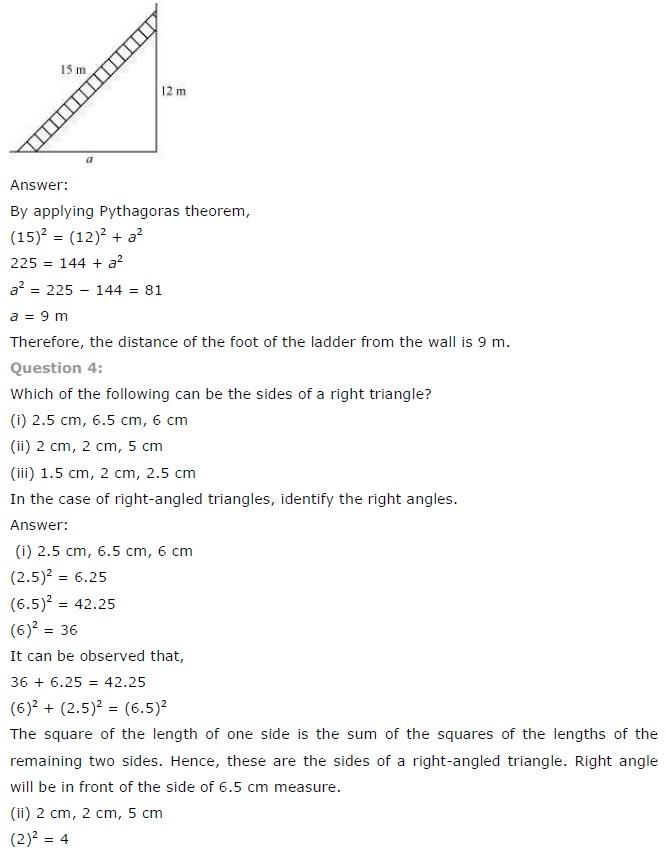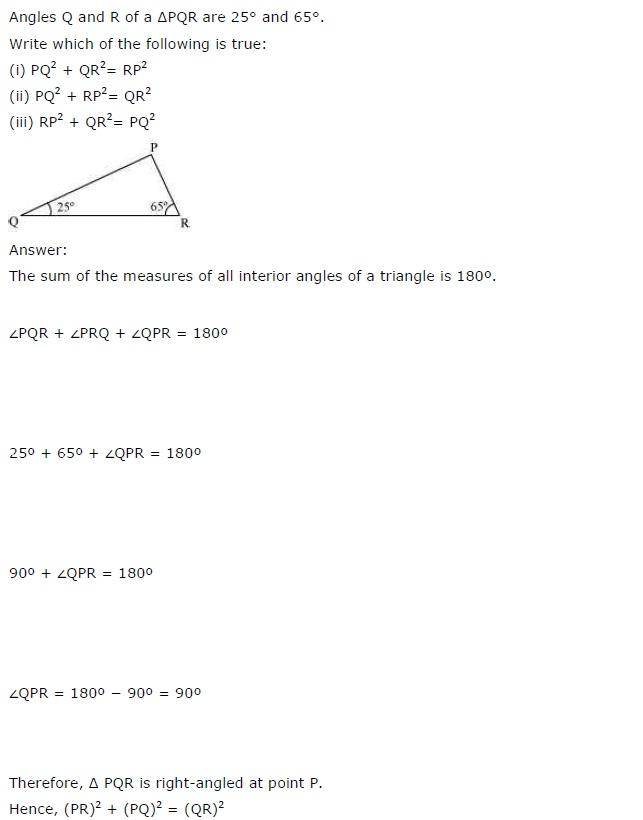Hence,

Option (ii) is true

Question 7:
Find the perimeter of the rectangle whose length is 40 cm and a diagonal is 41 cm.

Here,
It is given in the question that,

Length of a rectangle = 40 cm
Diagonal of a rectangle = 41 cm
We know that,
Diagonal of a rectangle divides it into two right angled triangles
By using Pythagoras theorem, we get
(41)2 = (40)2 + b2
b2 = (41)2 – (40)2
b2 = 1681 – 1600
b2 = 81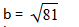b = 9 cm
Thus,
Perimeter of rectangle = 2 (l + b)
= 2 (40 + 9)
= 2 (49)
= 98 cm

Question 8:
The diagonals of a rhombus measure 16 cm and 30 cm.  Find its perimeter.